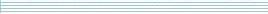| home      | people     | research     | publications    | seminars    | events     | contactCommunication Networks   | Systems Biology   | Hybrid Systems    | Machine Learning   | Dynamics & Interaction### Schools Mathematics Grand Challenge

Week one's Puzzles

Problem 1:

The first problem was:

You have two squares. The lengths of their sides are whole numbers of cm. The total area of the two squares is 34 square cm. If you make a rectangle using two sides from one square and two sides from the other, what would its area be?

The solution was:

The first thing to notice is that 34 can only be written as the sum of two squares of positive whole numbers in one way.

34 = (3 x 3) + (5 x 5).
This means that the sides of the two squares are 3cm and 5cm respectively. If we now make a rectangle with the short side of length 3cm and the long side of length 5cm, its area will be 15 square cm.

You could also form a rectangle with four sides of length (3+5), or one with two of length (3+3) and two of length (5+5). We have accepted the answers 60 and 64 square cm for this reason.

Problem 2:

The second problem was:

A teacher picks two consecutive whole numbers between 1 and 5. She tells John what one of the numbers is and tells Sheila the other one. John and Sheila then have the following conversation:

John: I do not know your number.
Sheila: I do not know your number.

What number was given to Sheila?

Hint: consecutive numbers are beside one another, so 8 and 9 are consecutive but 10 and 12 are not.

The solution was:

The numbers are consecutive so if John has either 1 or 5, he would immediately know Sheila's number. So John cannot have 1 or 5. Knowing that John's number must be 2, 3 or 4 and that the numbers are consecutive, the only way that Sheila would NOT know John's number is if her number is 3. So the answer is 3.

Problem 3:

The thrid problem was:

Back to wine gums! A bag contains 28 red, 31 green, 36 black, 29 orange and 27 yellow wine gums. Michael has been blindfolded so that he cannot see into the bag. What is the least number of wine gums that Michael must choose from the bag to guarantee that he has chosen at least THREE of the same colour?

The solution was:

There are only five different colours. If we choose 6 wine gums, then we must have 2 of the same colour. In order to guarantee that we also choose another wine gum of this colour, we need to pick another 5 wine gums. Hence the answer is 6+5 = 11.